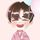cancel
Showing results for
Did you mean:Frequent Visitor

## Wrong table total

Hello!
I reach out to you in hopes to get help here.

I have tables where the total does not sum up the correct way. See example:The red area to the left= The companies in our business.

As you can see I have highlited the total rows which is not sum up the correct way.
The "average lönekostnad ex soc" is not supposed to be in the table, but I brought it in here since I use it in the measure for "total lönekostnad ex soc". The total row in "Total lönekostnad ex soc" is multiplying "antal konsulter"=208 x "Average lönekostnad ex soc"=-45001,75.
This is not correct. I want the "total lönekostnad ex soc" total row to summarize the total for the rows that is over it.

This is the measure I use for the "total lönekostnad ex soc":

Do you understand my problem?

Hope that you can help me!

Best regards!

1 ACCEPTED SOLUTIONCommunity Support

Hi @CApowerbi ,

I suggest you to use SUMX() function to create a new measure based on [Average lönekostnad ex soc].

``````New Average lönekostnad ex soc =
SUMX (
VALUES ( 'TableName'[ColumnName on Matrix Row] ),
)``````
``````Total lönekostnad ex. soc =
[New Average lönekostnad ex soc] * [Antal konsulter]``````

Best Regards,
Rico Zhou

If this post helps, then please consider Accept it as the solution to help the other members find it more quickly.Community Support

Hi @CApowerbi ,

I suggest you to use SUMX() function to create a new measure based on [Average lönekostnad ex soc].

``````New Average lönekostnad ex soc =
SUMX (
VALUES ( 'TableName'[ColumnName on Matrix Row] ),
)``````
``````Total lönekostnad ex. soc =
[New Average lönekostnad ex soc] * [Antal konsulter]``````

Best Regards,
Rico Zhou

If this post helps, then please consider Accept it as the solution to help the other members find it more quickly.